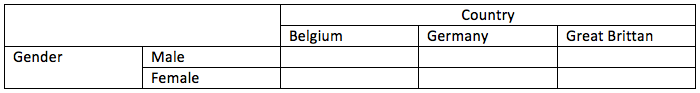MANOVA (Multivariate variance of analysis)# Research-Coaches.com

## We show you the way how to conduct perfect research

### MANOVA stands for Multivariate ANalysis Of VAriance. With this statistical technique, the scores of one variable are distributed among different groups. The means of the groups are compared with each other.

A MANOVA is an extension of an ANOVA. In the ANOVA the scores of the subjects are divided among three or more groups. For each groups the mean is computed and the deviations between the means is tested. The details can be found on our webpage called ANOVA.

In a MANOVA the scores of the cases are divided over two or more factors. For instance, the earnings of people can be compared among several countries and in the same analysis for gender too. This creates a two-way contingency table, like this one:For every cell a mean is computed.

It is easy to extend this table with extra dimensions, for instance type of work or branch. It will make a multidimensional table. Take care not to create too many cells, because for every cell a minimum number of fifteen respondents or objects is required to allow you to use MANOVA. This has to do with the degrees of freedom for this test.

## Examples of research questions for a MANOVA

The MANOVA is applied with research questions like:

• Do male and female blackbirds, starlings and seagulls differ in size?
• Do men and women in Italy, Great Brittan, Germany and Spain earn different amounts of money for same types of work?
• Is there a difference between, Africa, America, Asia, Australia and Europe in the amount of CO2-emission per type of industry?

## The hypothesis for testing the MANOVA

In an ANOVA there is only one grouping variable. It means there is only one dimension and on this dimension the means are compared. In a MANOVA there are (at least) two dimensions, and every dimension is tested separately. However, there is an extra dimension to be tested: the combination of both dimensions. This is called the interaction effect. It is written with a star between the names of the variables, for instance Country * Gender. In a two-way MANOVA three test are done: one for Country, one for Gender and one for Country * Gender. The first two are called main effects.

In a study with three dimensions already seven analyses are done. To show you how fast the number of tests expand, three variables are examined called A, B and C. Now these analyses are performed:

A Main effect of A

B Main effect of B

C. Main effect of C

two way interaction effect of A * B

two way interaction effect of A * C

two way interaction effect of B * C

three way interaction effect of A * B * C

With four dimensions (grouping variables A, B, C and D) already fifteen analyses are performed.

A

B

C

D

A * B

A * C

A * D

B * C

B * D

C * D

A * B * C

A * B * D

A * C * D

B * C * D

A * B * C * D

Now it might be clear to you why not too many grouping variables should be used. Not only a lot of cases, objects or respondents are needed, but it is also more difficult to interpret the result.

## Requirements for a MANOVA

Characteristic for an MANOVA is the comparing of the mean between classifications on at least two factors. The number of groups (the independent variables) should be at least two times two. The dependent variable should be a continuous variable, that is, it must be measured at an interval or ratio scale. If it is measured at an ordinal scale, there isn’t a widely used kind of analysis. The best way to deal with such a situation is treating the data as if they were measured at the interval level. Read more about this topic in our paper which test should be performed?

## Related topics to MANOVA